## Affine gap cost

If you believe that your data contains relatively few gaps, though they may be quite long, you can use the -G/--gapopen parameter to introduce an additional penalty for opening the gap in the first place. This would typically be used along with a low per-base gap cost, typically 1.

In this scheme the total cost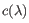of a gap of length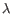is given by the following formula: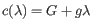(4.1)

where the open cost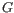and the extension cost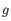are specified using -G and -g, respectively. Note that the affine model reduces to the linear one, when the open cost,, is set to zero.

Using a combination of a relatively high open cost and a low extension (per-base) gap cost, indicates to the mapper that you expect few gaps, but that these may be quite long.

You should be careful about using high open costs, if you have lots of sequencing errors in the form of deletions or insertions, as these may be penalized to the point, where you get lots of long unaligned ends. In such cases linear gap cost may be a better choice.

Like linear gap costs, affine gap costs can be used asymmetrically. This is done by independently setting the deletion open cost using the -E/--deletionopen parameter.